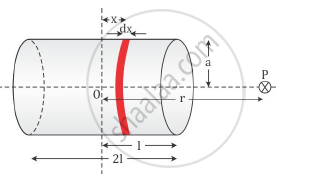# Derive the Expression for the Magnetic Field Due to a Solenoid - Physics

Derive the expression for the magnetic field due to a solenoid of length ‘2l’, radius ‘a’ having ’n’ number of turns per unit length and carrying a steady current ‘I’ at a point
on the axial line, distance ‘r’ from the centre of the solenoid. How does this expression compare with the axial magnetic field due to a bar magnet of magnetic moment ‘m’?

#### Solution

Consider a solenoid of length 2l, radius a and having n turns per unit length. It is carrying a current I.

We have to evaluate the axial field at a point P at a distance r from the centre of the solenoidConsider a circular element of thickness dx of the solenoid at a distance x from its centre.

The magnitude of the field due to this circular loop carrying a current I is given as

dB=(mu_0dxnIa^2)/(2[(r-x)^2+a^2]^(3/2))

The magnitude of the total field is obtained by integrating over all the elements from x = −l to x = +l.

B=(mu_0nIa^2)/2int_(-1)^(+1)dx/(2[(r-x)^2+a^2]^(3/2))

Consider the far axial field of the solenoid, so r ≫a and r≫l. Hence, we have

[(r-x)2+a2]3/2 ≈ r3

So, we get

B=(mu_0nIa^2)/(2r^3)int_(-1)^(+1)dx

B=(mu_0nIa^2)/(2r^3)2l

The magnitude of the magnetic moment of the solenoid is

m Total number of turns current cross-sectional area

m=(nx2l)xIx(πa2)

Therefore, we get the magnetic field as

B=(mu_0)/(4pi) (2m)/r^3

This is the expression for magnetic field due to a solenoid on the axial line at a distance r from the centre.

This magnetic field is also the field due to a bar magnet of magnetic moment m.

Concept: Solenoid and the Toroid - the Solenoid
Is there an error in this question or solution?
2014-2015 (March) Panchkula Set 3

Share